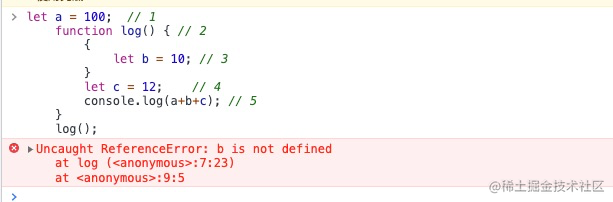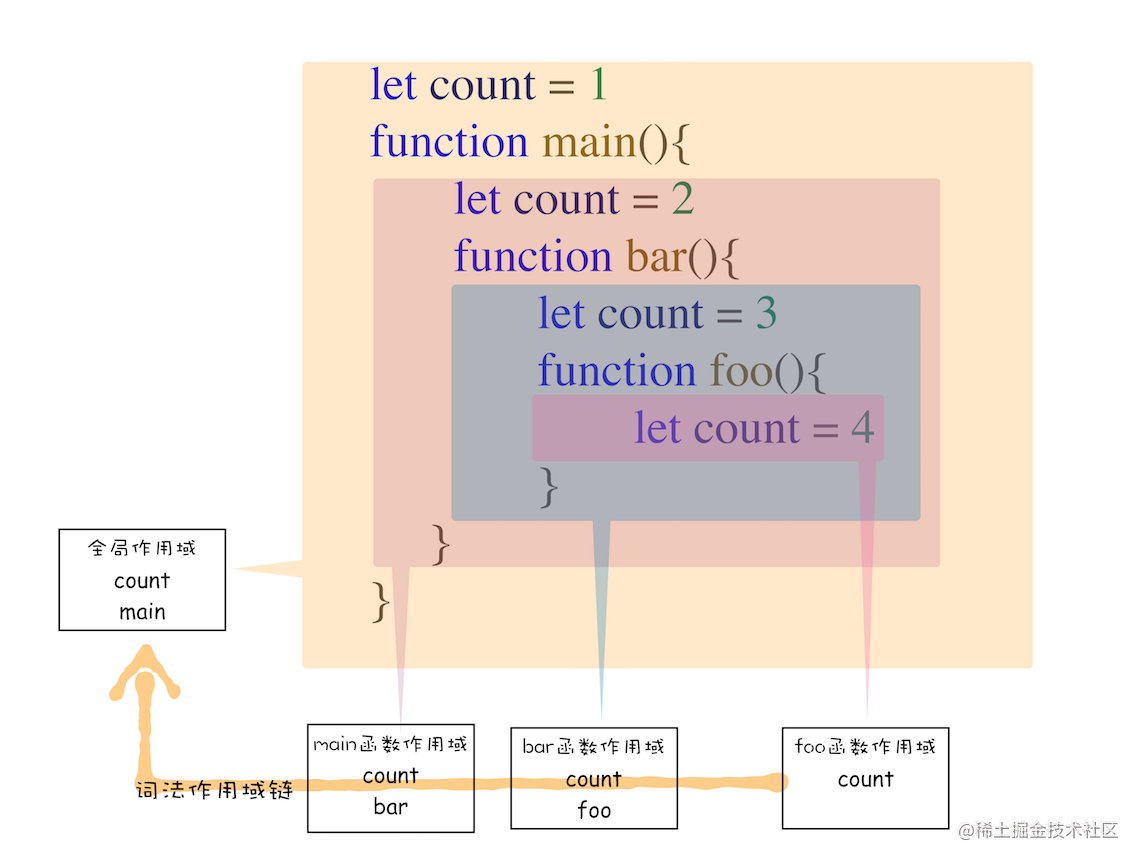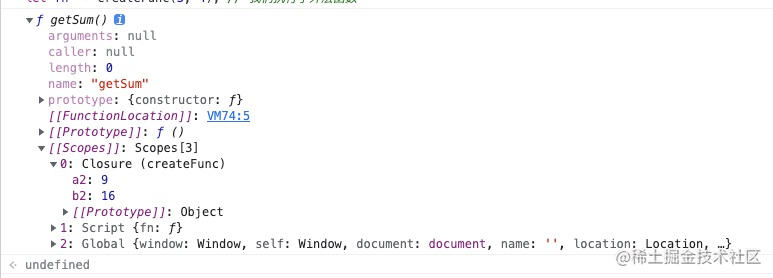再谈JS中的闭包先说观点

2. 产生闭包的条件

• 要素1：两个函数有嵌套关系，即在 一个函数中，定义另一个函数
• 要素2：内部函数是在外部函数执行结束后才执行。 表现形式可以是，内部函数做为返回值返回
• 要素3：内部函数内访问了外部函数中的变量。 可以是实参或内部声明的变量

function createFunc(a, b) {
let a2 = a*a;
let b2 = b*b;

return function () {
return a2+b2; // 访问外层函数的局部变量
}

}

let fn  = createFunc(3, 4); // 我们执行了外层函数
fn() // 输出 25

function sumsqu(a, b) {

let fn = function() {
return a*a + b*b;
}
return fn();
}

sumsqu(3, 4) // 25

再深入一点

1. 作用域

let a = 100;  // 1
function log() { // 2
{
let b = 10; // 3
}
let c = 12;    // 4
console.log(a+b+c); // 5
}
log();

1. 这段代码中我们 定义了a 和 函数 log 他们是位于全局作用域中的，可以把全局作用域 想象成一个大的盒子
2. 最后一行我们调用了 log 函数 ，会进行一个标识符查找 ，因为这个log()位于代码最外层， 所以他的查找的范围是 全局作用域，可以很快的找到 函数 log
3. 下面执行流程进入 log 函数内部 ，在 3 的地方 我们定义了一个 b = 10 ,它位于一对大括号中，这样会形成一个新的作用域(块级作用域)，就是就是一个新的小盒子，这个盒子中，只有一个 b , 这个 b 对盒子外的代码 是不可见的
4. 在 4 的地方， 我们定义了一个变量 c ，很明显，他定义在 函数log 作用域内, c的可访问范围就是这个函数
5. 在 5 的地方，稍微有一点复杂，首先这是一个函数调用语句 ，js引擎要先查找 console 标识符，在 函数 log 中并没有出现过，于是 js 引擎会向上查找，进入全局作用域， 在全局作用域 console 是内置对象，直接返回，接着继续查找 log 属性，也成功找到。 a 的 查找过程与console 类似
6. 注意 b 是无法找到的，这段代码最终是会报错，因为标识符查找，只会向上级查找2. 静态作用域与作用域链1. 我们把这种基于代码结构分析 得到作用域的机制，叫 静态作用域也叫 词法作用域， 它是由JS引擎，按ECMAScript-262 标准来实现的
2. 我们把 作用域嵌套 和 标识符由内向外的查找规则 ，叫 作用域链， 也就是标识符的每次查找都会由内向外在作用域链上查找

3. 静态作用域与闭包的关系

function createFunc(a, b) {
let a2 = a*a;
let b2 = b*b;

function getSum() {
return a2+b2; // 访问外层函数的局部变量
}

console.dir(getSum) // 打印 函数  getSum

return getSum;

}

let fn  = createFunc(3, 4); // 我们执行了外层函数
fn() // 输出 25

1. 这块代码中有两个作用域 createFunc 函数 作用域，和 getSum 函数作用域，两个作用域相互嵌套
2. getSum 函数中访问了 外层作用域中的 a2b2 两个变量
3. 依据 作用域链的规则 ，在函数getSum 中 就应该可以访问 a2b2
4. createFunc 执行结束后，getSum 并没有执行
5. 一般来讲，函数中的变量，会在函数执行完成后，释放掉！但有一种情况例外
6. 为了实现第三点，我们必需找一个地方把 a2b2 存在起来，在函数 getSum 调用时使用，只有这样才符合 JavaScript 静态作用域的规则1. 闭包的是在对 函数进行 词法分析 时创建的，为了节约内存，闭包中只保留必要的值，也就是在这个函数执行时要用到的变量！

闭包的运用

1. 防抖和节流

// 防抖
function debounce(fn, delay = 300) {
let timer;
return function () {
const args = arguments;
if (timer) {
clearTimeout(timer);
}
timer = setTimeout(() => {
fn.apply(this, args);
}, delay);
};
}

// 节流
function throttle(fn, delay) {
let flag = true;
return function () {
let args = arguments;
if (!flag) return;
flag = false;
timer = setTimeout(() => {
fn.apply(this, args);
}, delay);
};
}

2. 生成连续ID

let uniqueId = function() {
let start = 0;
return function (prefix = 'id_') {
let id = ++start;
if(prefix === 'id_') {
return  `\${id}`;
}
return `\${prefix}\${id}`
}
}();

总结

1. 闭包的本质是一组没有释放的变量（或内存区域），并且在函数被执行时，加到入执行上下文中！
2. 闭包产生原因：因为 JavaScript 遵循静态作用域规则, 为了保证函数在执行时可以访问外层作用域的变量，而形成的一种实现机制

参考

1. 《你不知道的 JavaScript 上》
2. Closures - JavaScript | MDN
3. 面试官：说说作用域和闭包吧
4. JavaScript 的静态作用域链与“动态”闭包链